# Quadrilaterals NCERT Solutions - Mathematics (Maths) Class 9

## Exercise 8.1

Q1. If the diagonals of a parallelogram are equal, then show that it is a rectangle.
Ans: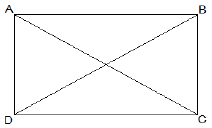Given that,
AC = BD
To show that ABCD is a rectangle if the diagonals of a parallelogram are equal
To show ABCD is a rectangle, we have to prove that one of its interior angles is right-angled.
Proof,
AB = BA (Common)
BC = AD (Opposite sides of a parallelogram are equal)
AC = BD (Given)
Therefore, ΔABC ≅ ΔBAD [SSS congruency]
∠A = ∠B [Corresponding parts of Congruent Triangles]
also,
∠A+∠B = 180° (Sum of the angles on the same side of the transversal)
⇒ 2∠A = 180°
⇒ ∠A = 90° = ∠B
Therefore, ABCD is a rectangle.
Hence Proved.

Q2. Show that the diagonals of a square are equal and bisect each other at right angles.
Ans: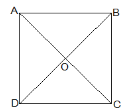Let ABCD be a square and its diagonals AC and BD
intersect each other at O.
To show,
AC = BD, AO = OC and ∠AOB = 90°
Proof,
BC = BA (Common)
Therefore, ΔABC ≅ ΔBAD by SAS congruence condition.
Thus, AC = BD by CPCT. Therefore, diagonals are equal.
Now,
In ΔAOB and ΔCOD,
∠BAO = ∠DCO (Alternate interior angles)
∠AOB = ∠COD (Vertically opposite)
AB = CD (Given)
Therefore, ΔAOB ≅ ΔCOD by AAS congruence condition.
Thus, AO = CO by CPCT. (Diagonal bisect each other.)
Now,
In ΔAOB and ΔCOB,
OB = OB (Given)
AO = CO (diagonals are bisected)
AB = CB (Sides of the square)
Therefore, ΔAOB ≅ ΔCOB by SSS congruence condition.
also, ∠AOB = ∠COB
∠AOB + ∠COB = 180° (Linear pair)
Thus, ∠AOB = ∠COB = 90° (Diagonals bisect each other at

Q3. Diagonal AC of a parallelogram ABCD bisects ∠A (see Fig.). Show that
(i) it bisects ∠C also,
(ii) ABCD is a rhombus.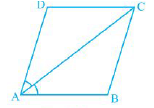Ans:
AD = CB (Opposite sides of a ||gm)
DC = BA (Opposite sides of a ||gm)
AC = CA (Common)
Therefore, ΔADC ≅ ΔCBA by SSS congruence condition.
Thus,
∠ACD = ∠CAB by CPCT
⇒ ∠ACD = ∠BCA
Thus, AC bisects ∠C also.

⇒ AD = CD (Opposite sides of equal angles of a triangle are
equal)

Also, AB = BC = CD = DA (Opposite sides of a ||gm)
Thus, ABCD is a rhombus.

Q4. ABCD is a rectangle in which diagonal AC bisects ∠A as well as ∠C. Show that:
(i) ABCD is a square
(ii) diagonal BD bisects ∠B as well as ∠D.

Ans: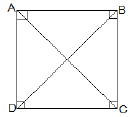(i)∠DAC = ∠DCA (AC bisects ∠A as well as ∠C)
⇒ AD = CD (Sides opposite to equal angles of a triangle are equal) also, CD = AB (Opposite sides of a rectangle)
Therefore, AB = BC = CD = AD
Thus, ABCD is a square.

(ii) In ΔBCD,
BC = CD
⇒ ∠CDB = ∠CBD (Angles opposite to equal sides are equal)
also, ∠CDB = ∠ABD (Alternate interior angles)
⇒ ∠CBD = ∠ABD
Thus, BD bisects ∠B
Now,
Thus, BD bisects ∠D

Q5. In parallelogram ABCD, two points P and Q are taken on diagonal BD such that DP = BQ (see Fig). Show that:
(i) ΔAPD ≅ΔCQB
(ii) AP = CQ
(iii) ΔAQB ≅ ΔCPD
(iv) AQ = CP
(v) APCQ is a parallelogram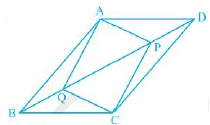Ans:
(i) In ΔAPD and ΔCQB,
DP = BQ (Given)
∠ADP = ∠CBQ (Alternate interior angles)
AD = BC (Opposite sides of a ||gm)
Thus, ΔAPD ≅ ΔCQB by SAS congruence condition.
(ii) AP = CQ by CPCT as ΔAPD ≅ ΔCQB.
(iii) In ΔAQB and ΔCPD,
BQ = DP (Given)
∠ABQ = ∠CDP (Alternate interior angles)
AB = BCCD (Opposite sides of a ||gm)
Thus, ΔAQB ≅ ΔCPD by SAS congruence condition.

(iv) AQ = CP by CPCT as ΔAQB ≅ ΔCPD.
(v) From (ii) and (iv), it is clear that APCQ has equal
opposite sides also it has equal opposite angles. Thus,
APCQ is a ||gm.

Q6. ABCD is a parallelogram and AP and CQ are perpendiculars from vertices A and C on diagonal BD (see Fig). Show that
(i) ΔAPB ≅ ΔCQD
(ii) AP = CQ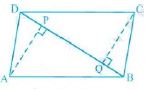Ans:
(i) In ΔAPB and ΔCQD,
∠ABP = ∠CDQ (Alternate interior angles)
∠APB = ∠CQD (= 90o as AP and CQ are perpendiculars)
AB = CD (ABCD is a parallelogram)
ΔAPB ≅ ΔCQD [AAS congruency]
(ii) As ΔAPB ≅ ΔCQD. AP = CQ [CPCT]

Q7. ABCD is a trapezium in which AB || CD and AD = BC (see Fig). Show that
(i) ∠A = ∠B
(ii) ∠C = ∠D
(iv) diagonal AC = diagonal BD
[Hint : Extend AB anddraw a line through C parallel to DA intersecting AB produced at E.]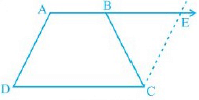Ans: To Construct: Draw a line through C parallel to DA intersecting AB produced at E.
(i) CE = AD (Opposite sides of a parallelogram)
, BC = CE
⇒∠CBE = ∠CEB
also,
∠A+∠CBE = 180° (Angles on the same side of transversal and ∠CBE = ∠CEB)
∠B +∠CBE = 180° ( As Linear pair)
⇒∠A = ∠B
(ii) ∠A+∠D = ∠B+∠C = 180° (Angles on the same side of transversal)
⇒∠A+∠D = ∠A+∠C (∠A = ∠B)
⇒∠D = ∠C
AB = AB (Common)
∠DBA = ∠CBA
, ΔABC ≅ ΔBAD [SAS congruency]
(iv) Diagonal AC = diagonal BD by CPCT as ΔABC ≅ ΔBAD.

## Exercise 8.2

Q1. ABCD is a quadrilateral in which P, Q, R and S are mid-points of the sides AB, BC, CD and DA (see Fig). AC is a diagonal.
Show that:
(i) SR || AC and SR = (1/2) AC
(ii) PQ = SR
(iii) PQRS is a parallelogram.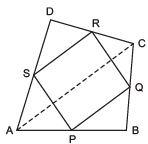Ans: We have P as the mid-point of AB, Q as the mid-point of BC, R as the mid-point of CD, S as the mid-point of DA, and AC as the diagonal of quadrilateral ABCD.
(i) To prove that SR =(1/2) AC and SR || AC.
In ΔACD, we have
S as the mid-point of AD, R as the mid-point of CD.
∵ The line segment joining the mid-point of any two sides of a triangle is parallel to the third side and half of it.
∴ SR = (1/2)AC and SR || AC
(ii) To prove that PQ = SR.
In ΔABC, we have P is the mid-point of AB, Q is the mid-point of BC.
∴ PQ = (1/2) AC            …(1)
Also, SR = (1/2) AC            [Proved]             …(2)
From (1) and (2), PQ = SR
(iii) To prove that PQRS is a parallelogram.
In ΔABC, P and Q are the mid-points of AB and BC.
∴ PQ = (1/2)AC and PQ || AC             ...(3)
In ΔACD, S and R are the mid-points of DA and CD.
∴ SR = (1/2)AC and SR || AC             …(4)
From (3) and (4), we get
PQ =(1/2)AC = SR and PQ || AC || SR
⇒ PQ = SR and PQ || SR
i.e. One pair of opposite sides in quadrilateral PQRS is equal and parallel.
∴ PQRS is a parallelogram.

Q2. ABCD is a rhombus and P, Q, R and S are the mid-points of the sides AB, BC, CD and DA respectively. Show that the quadrilateral PQRS is a rectangle.
Ans: We have P as the mid-point of AB, Q as the midpoint of BC, R as the mid-point of CD, S as the mid-point of DS.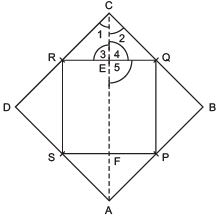We have to prove that PQRS is a rectangle.
Let us join AC.
∵ In ΔABC, P and Q are the mid-points of AB and BC.
∴ PQ =(1/2)AC and PQ || AC             …(1)
Also in ΔADC, R and S are the mid-points of CD and DA.
∴ SR = (1/2)AC and SR || AC
From (1) and (2), we get
PQ =(1/2) AC = SR and PQ || AC || SR
⇒ PQ = SR and PQ || SR
i.e. One pair of opposite sides of quadrilateral PQRS is equal and parallel.
∴ PQRS is a parallelogram.
Now, in ΔERC and ΔEQC, ∠1= ∠2            [∵ The diagonal of a rhombus bisects the opposite angles]
CR = CQ            [Each is equal to(1/2) of a side of rhombus]CE = CE [Common]
∴ ΔERC ≌ ΔEQC            [SAS criteria]
⇒ ∠3= ∠4[c.p.c.t.]
But ∠3 + ∠4 = 180º [Linear pair] ⇒ ∠3= ∠4 = 90°
But ∠5= ∠3            [Vertically opposite angles]
∴ ∠5 = 90º PQ || AC
⇒ PQ || EF
∴ PQEF is a quadrilateral having a pair of opposite sides parallel and one of the angles is 90º.
∴ PQEF is a rectangle.
⇒ ∠RQP = 90º
∴ One angle of parallelogram PQRS is 90º.
Thus, PQRS is a rectangle.

Question 3. ABCD is a rectangle and P, Q, R and S are mid-points of the sides AB, BC, CD and DA respectively. Show that the quadrilateral PQRS is a rhombus.
Ans: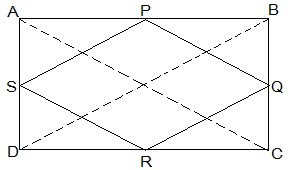Given in the question,
ABCD is a rectangle and P, Q, R and S are mid-points of the sides AB, BC, CD and DA, respectively.
Construction,
Join AC and BD.
To Prove,
PQRS is a rhombus.
Proof:
In ΔABC
P and Q are the mid-points of AB and BC, respectively
, PQ || AC and PQ = ½ AC (Midpoint theorem) — (i)
SR || AC and SR = ½ AC (Midpoint theorem) — (ii)
So, PQ || SR and PQ = SR
As in quadrilateral PQRS one pair of opposite sides is equal and parallel to each other, so, it is a parallelogram.
, PS || QR and PS = QR (Opposite sides of parallelogram) — (iii)
Now,
In ΔBCD,
Q and R are mid points of side BC and CD, respectively.
, QR || BD and QR = ½ BD (Midpoint theorem) — (iv)
AC = BD (Diagonals of a rectangle are equal) — (v)
From equations (i), (ii), (iii), (iv) and (v),
PQ = QR = SR = PS
So, PQRS is a rhombus.
Hence Proved

Q4. ABCD is a trapezium in which AB || DC, BD is a diagonal and E is the mid-point of AD. A line is drawn through E parallel to AB intersecting BC at F (see Fig). Show that F is the mid-point of BC.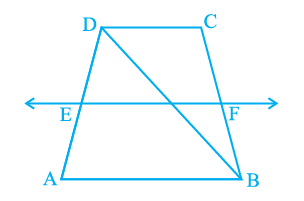Ans: Given that,
ABCD is a trapezium in which AB || DC, BD is a diagonal and E is the mid-point of AD.
To prove,
F is the mid-point of BC.
Proof,
BD intersected EF at G.
E is the mid point of AD and also EG || AB.
Thus, G is the mid point of BD (Converse of mid point theorem)
Now,
In ΔBDC,
G is the mid point of BD and also GF || AB || DC.
Thus, F is the mid point of BC (Converse of mid point theorem)

Q5. In a parallelogram ABCD, E and F are the mid-points of sides AB and CD respectively (see Fig). Show that the line segments AF and EC trisect the diagonal BD.
Ans: We have ABCD is a parallelogram such that: E is the mid-point of AB and F is the mid-point of CD.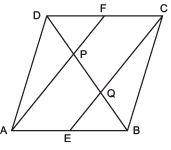Let us join the opposite vertices B and D.
Since, the opposite sides of a parallelogram are parallel and equal.
∴ AB || DC ⇒ AE || FC            …(1)
Also AB = DC
or (1/2) AB =(1/2)DC ⇒ AE = FC            …(2)
From (1) and (2),
we can say that AECF is quadrilateral having a pair of the opposite sides as parallel and equal.
∴ AEFC is a parallelogram.
⇒ AE || CF
Now, in DABC, F is the mid-point of DC             [Given]
and FP || CQ             [∵ AF || CE]
⇒ P is the mid-point of DQ             [Converse of mid-point theorem]
⇒ DP = PQ             …(3)
Similarly, in DBAP, BQ = PQ             …(4)
∴ From (3) and (4), we have
DP = PQ = BQ
⇒ The line segments AF and EC trisect the diagonal BD.

Q6. ABC is a triangle right angled at C. A line through the mid-point M of hypotenuse AB and parallel to BC intersects AC at D. Show that
(i) D is the mid-point of AC
(ii) MD ⊥ AC
(iii) CM = MA = 1/2 AB
Ans: We have a triangle ABC, such that ∠C = 90º M is the mid-point of AB and MD || BC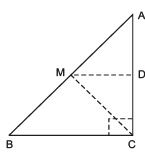(i) To prove that D is the mid-point of AC.
In ΔACB, we have M as the mid-point of AB.             [Given]
MD || BC            [Given]
∴ Using the converse of mid-point theorem, D is the mid-point of AC.
(ii) To prove that MD ⊥ AC.
Since, MD || BC             [Given] and AC is a transversal.
∴ ∠ MDA = ∠BCA            [Corresponding angles]
But ∠BCA = 90º            [Given]
∴ ∠MDA = 90º ⇒ MD ⊥ AC.
(iii) To prove that CM = MA =(1/2) AB
In ΔADM and ΔCDM, we have
∠ADM = ∠CDM            [Each = 90º]
MD = MD            [Common]
AD = CD            [∵ M is the mid-point of AC (Proved)]
∴ ΔADM ≌ ΔCOM            [SAS criteria]
⇒ MA = MC [c.p.c.t.]             …(1)
∵ M is the mid-point AB.            [Given]
∴ MA =(1/2)AB              …(2)
From (1) and (2), we have
CM = MA = (1/2) AB

The document Quadrilaterals NCERT Solutions | Mathematics (Maths) Class 9 is a part of the Class 9 Course Mathematics (Maths) Class 9.
All you need of Class 9 at this link: Class 9

## Mathematics (Maths) Class 9

42 videos|378 docs|65 tests

## FAQs on Quadrilaterals NCERT Solutions - Mathematics (Maths) Class 9

 1. What are the different types of quadrilaterals?Ans. There are several types of quadrilaterals, including parallelograms, rectangles, squares, rhombuses, trapeziums, and kites.
 2. What are the properties of a parallelogram?Ans. A parallelogram has opposite sides that are parallel and congruent, opposite angles that are congruent, and diagonals that bisect each other.
 3. How do you determine if a quadrilateral is a rectangle?Ans. To determine if a quadrilateral is a rectangle, you need to check if it has all four right angles. If the opposite sides are parallel and congruent as well, then it is a parallelogram.
 4. What is the difference between a square and a rhombus?Ans. A square is a special type of rhombus where all four sides are equal in length and all four angles are right angles. A rhombus, on the other hand, has all four sides equal in length but its angles are not necessarily right angles.
 5. How do you find the area of a trapezium?Ans. The area of a trapezium can be found by multiplying the sum of the lengths of its parallel sides (the bases) by its height and dividing the result by 2. The formula is: Area = (base1 + base2) × height / 2.

## Mathematics (Maths) Class 9

42 videos|378 docs|65 testsExplore Courses for Class 9 examSignup to see your scores go up within 7 days! Learn & Practice with 1000+ FREE Notes, Videos & Tests.
10M+ students study on EduRev
Track your progress, build streaks, highlight & save important lessons and more!
Related Searches

,

,

,

,

,

,

,

,

,

,

,

,

,

,

,

,

,

,

,

,

,

;